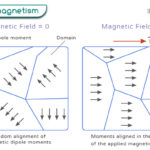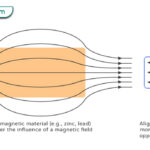Home / Physics / Tension

# Tension

## What is Tension

The tension or tension force is a force experienced by a rope, string, or cable when it is pulled from the opposite end. It acts along the length of the wire and is equal and opposite to the applied force, that is, the force with which it is pulled. In this way, the tension force balances the applied force. It is a contact force and passes through a flexible medium. Tension force is transmitted through a rope only when it is pulled. Otherwise, the action of pushing a rope results in a slack.

## Tension Force Examples

Here are a few examples of the tension force from everyday life.

• Suspension of a pendulum bob
• An object suspended by a pully
• Pulling a sleigh by huskies and reindeer
• Plucking a guitar string
• Stretching the muscles
• A swing in a playground
• Elevator suspended by a cable
• Spring in a watch
• Suspension bridge
• Seat belt

## Tension Formula

Suppose a force FA is applied to a rope. Then, the tension force T is given by,

T = FA

If a string suspends an object of mass m, then the tension is given by,

T = mg

Where, g is the acceleration due to gravity.

### How to Find the Tension Force

Suppose a block of mass m1 is lying on a horizontal table. Mass m2 pulls m1 using a pully as shown in the figure. The two blocks move with an acceleration a. According to Newton’s second law,

For m2: m2a = m2g – T

For m1: m1a = T

Solving for the acceleration,

a = m2g/(m1+m2)

By using the expression for a in the second equation above, one can obtain the following.

Tension Force Equation: T = m1m2g/(m1+m2)

Unit for Tension: Newton or N

If m1 = m2 = m,

T = mg/2

## Tension Problems

Problem 1: An object of mass 12 kg is suspended by light and an inextensible string at one end. If the string is attached firmly to a hook at the other end, calculate the force of tension.

Soln.: Since a string suspends the object, the only force acting on the body is its weight. The string has to bear a tension force equal to the weight to balance it.

T = mg = 12 kg x 9.81 m/s2 = 118 N

Problem 2: An object of mass 8 kg is pulled by a rope over a pulley. It is accelerating upward with an acceleration of 6 m/s2. Calculate the force of tension on the rope.

Soln.: Since the object is pulled upward, the tension force includes its weight and force due to its acceleration.

T = mg + ma = 8 kg x 9.81 m/s2+ 8 kg x 6 m/s2 = 126.5 N

## Tension vs. Compression

The following table compares and contrasts tension and compression.

Article was last reviewed on Tuesday, December 15, 2020

### Related articlesWave-Particle DualityPhotoelectric EffectFerromagnetismDiamagnetism

### One response to “Tension”

1. Geresu Gurme says:

GOD BLESS YOU